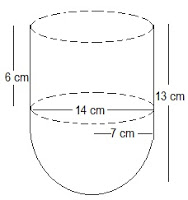# A vessel is in the form of a hollow hemisphere mounted by a hollow cylinder. The diameter of the hemisphere is 14 cm and the total height of the vessel is 13 cm. Find the inner surface area of the vessel.The diameter of the hemisphere = D = 14 cm

The radius of the hemisphere = r = 7 cm

The height of the cylinder = h = (13-7) = 6 cm

The radius of the hollow hemisphere = 7 cm

Inner surface area of vessels=C.S.A of cylinder+ C.S.A of Hemisphere

=2πrh+2πr2

=2πr(h+r)

=2*22/7*7(6+7)

=44(13)

=572cm2